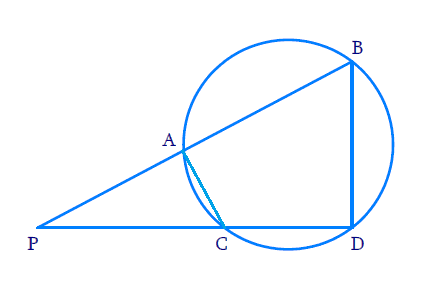# Ex. 6.6 Q8 Triangles Solution - NCERT Maths Class 10

Go back to  'Ex.6.6'

## Question

In Fig. below, two chords $$AB$$ and $$CD$$ of a circle intersect each other at the point $$P$$ (when produced) outside the circle.

Prove that:

(i) $$\Delta {\text{ }}PAC{\text{ }}\text{~}{\text{ }}\Delta {\text{ }}PDB$$

(ii) $$PA. PB = PC. PD$$Video Solution
Triangles
Ex 6.6 | Question 8

## Text Solution

Reasoning:

(i) Exterior angle of a cyclic quadrilateral is equal to the opposite interior angle.

Steps:

Draw $$AC$$

(i) In, $$\Delta PAC,\,\,\Delta PDB$$

\begin{align} & \angle APC=\angle BPD\,\,\, \\ & (\text{Common}\,\text{angle}) \\ & \\ & \angle PAC=\angle PDB\,\, \\ & \left( \begin{array} & \text{Exterior angle of a cyclic} \\ \,\text{quadrilateral is equal to the} \\ \text{interior opposite angle} \\ \end{array} \right)\, \\ & \\ & \Rightarrow \Delta PAC~\sim \Delta \text{PDB}\, \\ \end{align}

(ii) In, $$\Delta PAC,\,\,\Delta PDB$$

\begin{align} &\frac{{PA}}{{PD}} = \frac{{PC}}{{PB}} = \frac{{AC}}{{BD}}\\ &\frac{{PA}}{{PD}} = \frac{{PC}}{{PB}}\\ &PA \cdot PB = PC \cdot PD \end{align}

Learn from the best math teachers and top your exams

• Live one on one classroom and doubt clearing
• Practice worksheets in and after class for conceptual clarity
• Personalized curriculum to keep up with school